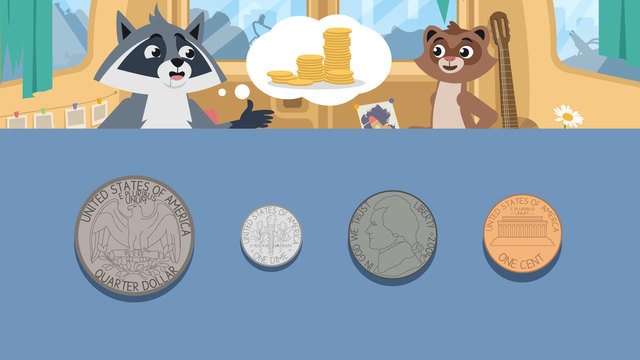Rating

Ø 4.4 / 5 ratings
The authorsTeam Digital
CCSS.MATH.CONTENT.2.MD.C.8

## Basics on the topicAdding Coins

Your parents gave you their wallet so you can buy yourself ice cream. But how many coins do you need for one scoop of ice cream? Let’s learn more about counting coins and how to count coins for beginners with the following explanation. At the end, don’t miss out on the counting coins interactive exercises, worksheets and more activities for 2nd grade.

## Coins – A Review

Remember, coins are little, flat, circular pieces of money made from metal. When counting mixed coins, it is important to remember that each coin has a different face, a different size, and a different value. When counting American coins, they are usually worth cents, or less than a dollar. We use THIS symbol, which looks like a “c” with a line through it, it represents cents.The values of US coins are as follows:Coin Worth
1 penny 1 cents
1 nickel 5 cents
1 dime 10 cents
1 quarter 25 cents

To review how to teach coins to second graders, let’s start with an example to model. There are several strategies for counting coins. Let's use the coins below (one penny, one dime, two quarters, and three nickels) to review this strategy for counting coins. First, order the coins from greatest to least by writing the value of each one below it.The order will be two quarters at twenty five cents each, one dime at ten cents, three nickels at five cents each and one penny at one cent.Next, set up an equation by adding all the values together.Then, solve the equation by adding up the values.Finally, put a cent symbol next to the answer since this represents money.## Counting Coins – Summary of Steps

Remember to follow these steps when counting coins:

Step # What to do
1 Order them from greatest to least
by writing their values beneath.